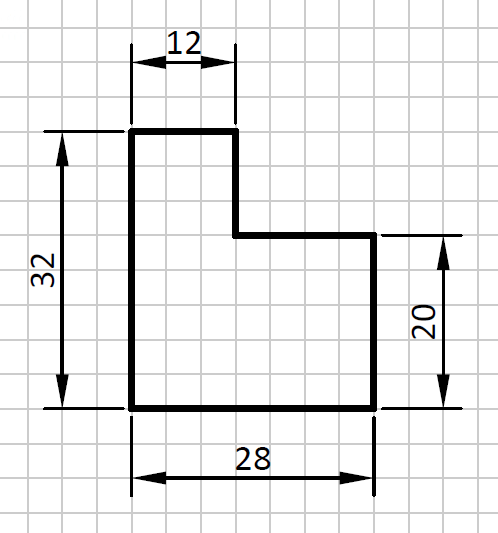# Dimension¶

To dimension is to add to the drawing the size of the real object. If an object is 20 millimeters in size, that amount will be represented in your drawing.The dimension is composed of several elements:

• Auxiliary dimension lines: 2 segments perpendicular to what is being measured, which determine the beginning and end of the measurement.
• Dimension line and arrows: line parallel to the dimension to be dimensioned, which measures the same and begins and ends with two dimension arrows.
• Dimension figure: number that indicates the size of the dimension, in millimeters if no other unit is specified.

If the drawing is larger than the object (enlargement) or smaller (reduction), the dimension always has the same value because it does not depend on the size of the drawing. The dimension represents the size of the actual object, not the size of the drawing.

## Dimensioning exercises¶

Below is a sheet with part dimensioning exercises. On the back of the sheet are the solved exercises.